# Machine Learning: Support Vector Machine

## Introduction

In the previous chapter, we studied the k-nearest neighbors.

In this chapter, we will learn support vector machine.

Note: ifyou can correlate anything with yourself or your life, there are greater chances of understanding the concept. So try to understand everything by relating it to humans.

## What is Support Vector Machine?

Support vector machines (SVMs, also supporting vector networks) in machine learning are supervised learning models with associated learning algorithms that analyze data used for classification and regression analysis. Provided a set of training instances, each classified as belonging to one or the other of two groups, a training algorithm SVM generates a template that sells new cases for one or the other group, which renders it a non-probabilistic linear binary classifier.

A model from SVM describes the examples as points in space and is distributed to separate the examples of the different groups through a very broad void. There are then projected new instances in the same area and a range dependent on the portion of the distance in which they fall is predicted.

A hyperplane or a number of hyperplanes in a small or infinite space may be created by the support- computer and can be used for the graduation or reversal or other tasks such as the identification of outliers. The hyperplanes with the largest distance from the closest training data point of any class (so-called functional margin) intuitively achieve a good separation, as the wider the margin, the lower the generalization error of the classification system.

Note:

When data are unlisted, supervised education is not available and there is a need for an unsupervised learning approach that attempts to find a natural grouping of data and then map new data to the groups that are formed.

## Key Terms

1. ### Kernel

• A kernel refers to a feature that converts the data into a wide space for solving the problem.
• A linear or non-linear kernel function may be used. Kernel methods are a type of pattern analysis algorithm.
• The kernel's primary role is to accept data as input and convert it into the appropriate output types.
• In statistics, the mapping feature "core" measures the values of a two-dominal data in a three-dimensional spatial format and describes them.

2. ### Regularization

• The regularization function is also named the C function in the sklearn library of python, which guides the help vector machine to optimally define each training date it wants to prevent.
• Such a supporting vector machine example will auto-optimization if large numbers of the C parameter are used if all training data points are correctly segregated and classification is collected the hyperplanes margin that is smaller.
• To order to obtain very tiny numbers, the algorithm can often consider that the hyperplane is a larger range and certain data points may be misclassified by the hyperplane.

3. ### Gamma

• This tuning function reiterates the length of the effect of a single data display. The low values are 'far' and the higher values are 'near' to the aircraft.
• In measurement for a separation line, the data points with low gamma are called and are far from the possible hyper-plane separation line.
• In comparison, the high range is used in the measurement of the hyper-plane separation line which applies to the points that are similar to the expected hyper-plane line.

4. ### Margin

• The gap is last but not least. It's also a significant tuning parameter & an integral function of a vector holder classification system.
• The margin is the division of the line closest to the data points of the segment. In a support vector algorithm, it is necessary to have a good and proper margin. When the difference between the two data groups is greater, a strong gap is called.
• To a strong range, the corresponding data points stay in class and thus do not move over to another level.
• Also, the class data points will have a reasonable margin preferably at the same distance from either side of the separator panel.

5. ### Hyperplane

• A hyperplane is a linear, n-1 dimensional subset of this space, whichsplits the space into two divided parts in an n-dimensional Euclidean space.
• For two dimensions the hyperplane is a separating line.
• For three dimensions a plane with two dimensions divides the 3d space into two parts and thus acts as a hyperplane.
• Thus for a space of n dimensions, we have a hyperplane of n-1 dimensions separating it into two parts.

## Types of SVM

1. Classification SVM type 1 (also known as C-SVM classification)
2. Classification SVM type 2 (also known as nu-SVM classification)
3. Regression SVM type 1 (also known as epsilon-SVM regression)
4. Regression SVM type 2 (also known as nu-SVM regression)

## Types of kernels

1. Linear kernel
2. Polynomial kernel
3. Radial basis function kernel (RBF)/ Gaussian Kernel
4. Sigmoid Kernel
5. Nonlinear Kernel

## Real-World Applications of SVM

1. Face detection

SVM classifies parts of the image as a face and non-face and creates a square boundary around the face.

2. Text and hypertext categorization

SVMs allow Text and hypertext categorization for both inductive and transductive models. They use training data to classify documents into different categories. It categorizes on the basis of the score generated and then compares with the threshold value.

3. Classification of images

Use of SVMs provides better search accuracy for image classification. It provides better accuracy in comparison to the traditional query-based searching techniques.

4. Bioinformatics

It includes protein classification and cancer classification. We use SVM for identifying the classification of genes, patients on the basis of genes, and other biological problems.

5. Protein fold and remote homology detection

Apply SVM algorithms for protein remote homology detection.

6. Handwriting recognition

We use SVMs to recognize handwritten characters used widely.

7. Generalized predictive control(GPC)

Use SVM based GPC to control chaotic dynamics with useful parameters.

## SVM using Example

Like in other ML algorithms we find the best fit, in SVM we try to find a hyperplane with the maximum margin or distance, hence it is also a type of "maximum-margin" classification.

Let us try to understand SVM with the help of a mathematical example, for this example,

 Data Points Class (-2, 4) -1 (4,1) -1 (1,6) 1 (2,4) 1 (6,2) 1

Now before I go on further I want you to first know:

### 1. Hinge loss

In machine learning, the hinge loss is a loss function used for training classifiers. The hinge loss is used for "maximum-margin" classification, most notably for support vector machines (SVMs).

The equation is given as: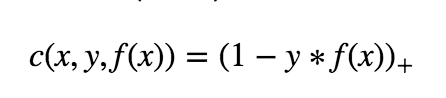where c is the loss function, x the sample, y is the true label, f(x) the predicted label.

You see a plus at the end, it means that the hinge error can never be negative, mathematically I can be expressed as: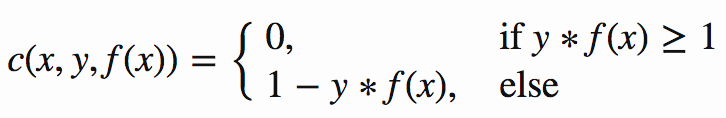### 2. Regularizer

The regularizer balances between margin maximization and loss. The regularizer controls the trade-off between achieving a low training error and a low testing error that is the ability to generalize your classifier to unseen data. As a regularizing parameter we choose 1/epochs, so this parameter will decrease, as the number of epochs increases.

Regularixer, λ = 1/epoch

### 3. Weight Vector

The SVM algorithm chooses a particular weight vector, that which gives rise to the “maximum margin” of separation

Now coming back to the math of SVM, generation of the hyperplane we can have 2 scenarios
1. Misclassification, i.e. the point is not classified correctly
2. Correct Classification

In the case of misclassification, we use the following to update the weights: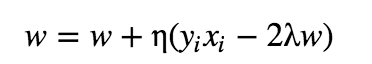and in case of correct classification, we use the following to update the weights: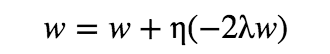where,
• η is the learning rate
• λ is the regularizer
After doing the calculations, I came up with the following prediction function:
f(x) = (x, (1.56, 3.17))- 11.12
i.e f(x) = 1.56*x + 3.17*y -11.12

where,
• (1.56, 3.17) is the weight vector
• 11.12 is the bias term
Note: I will not be going into depth on how I got this, you can do the calculations by yourself or you can use sklearn to do it for you.

Now let us check the accuracy of the prediction function so calculated

1. -2*1.56 + 4*3.17 - 11.12
= -1.56
taking the sign out, we get -1, which is the correct class

2. 4*1.56 + 1*3.17 - 11.12
= -1.72
taking the sign out, we get -1, which is the correct class

3. 1*1.56 + 6*3.17 - 11.12
= 9.46
taking the sign out, we get +1, which is the correct class

4. 2*1.56 + 4*3.17 - 11.12
= 4.68
taking the sign out, we get +1, which is the correct class

5. 6*1.56 + 2*3.17 - 11.12
= 4.58
taking the sign out, we get +1, which is the correct class

So until now, we tested the hyperplane equation on the training data. Now its time to give some never seen before data to the model

Test Data = (3, 5), (-2, 3)

1. 3*1.56 + 5*3.17 - 11.12
= 9.41
Taking the sign out, we get +1, which is the correct class

2. -2*1.56 + 3*3.17 - 11.12
= -4.73
Taking the sign out, we get -1, which is the correct class

## Python Implementation of SVM

### 1. Using Functions

Let us now take a look at how can we implement SVM from scratch. In the following example, we will take dummy data. I have taken the code reference from the repository.
1. # importing some basic libraries
2. %matplotlib inline
3. import matplotlib.pyplot as plt
4. from matplotlib import style
5. style.use('ggplot')
6. import numpy as np
7.
8. class SVM(object):
9.     def __init__(self,visualization=True):
10.         self.visualization = visualization
11.         self.colors = {1:'r',-1:'b'}
12.         if self.visualization:
13.             self.fig = plt.figure()
15.
16.     def fit(self,data):
17.         #train with data
18.         self.data = data
19.         # { |\w\|:{w,b}}
20.         opt_dict = {}
21.
22.         transforms = [[1,1],[-1,1],[-1,-1],[1,-1]]
23.
24.         all_data = np.array([])
25.         for yi in self.data:
26.             all_data = np.append(all_data,self.data[yi])
27.
28.         self.max_feature_value = max(all_data)
29.         self.min_feature_value = min(all_data)
30.         all_data = None
31.
32.         #with smaller steps our margins and db will be more precise
33.         step_sizes = [self.max_feature_value * 0.1,
34.                       self.max_feature_value * 0.01,
35.                       #point of expense
36.                       self.max_feature_value * 0.001,]
37.
38.         #extremly expensise
39.         b_range_multiple = 5
40.         #we dont need to take as small step as w
41.         b_multiple = 5
42.
43.         latest_optimum = self.max_feature_value*10
44.
45.         """
46.         objective is to satisfy yi(x.w)+b>=1 for all training dataset such that ||w|| is minimum
47.         for this we will start with random w, and try to satisfy it with making b bigger and bigger
48.         """
49.         #making step smaller and smaller to get precise value
50.         for step in step_sizes:
51.             w = np.array([latest_optimum,latest_optimum])
52.
53.             #we can do this because convex
54.             optimized = False
55.             while not optimized:
56.                 for b in np.arange(-1*self.max_feature_value*b_range_multiple,
57.                                    self.max_feature_value*b_range_multiple,
58.                                    step*b_multiple):
59.                     for transformation in transforms:
60.                         w_t = w*transformation
61.                         found_option = True
62.
63.                         #weakest link in SVM fundamentally
64.                         #SMO attempts to fix this a bit
65.                         # ti(xi.w+b) >=1
66.                         for i in self.data:
67.                             for xi in self.data[i]:
68.                                 yi=i
69.                                 if not yi*(np.dot(w_t,xi)+b)>=1:
70.                                     found_option=False
71.                         if found_option:
72.                             """
73.                             all points in dataset satisfy y(w.x)+b>=1 for this cuurent w_t, b
74.                             then put w,b in dict with ||w|| as key
75.                             """
76.                             opt_dict[np.linalg.norm(w_t)]=[w_t,b]
77.
78.                 #after w or w<0 then values of w starts repeating itself because of transformation
79.                 #Think about it, it is easy
80.                 #print(w,len(opt_dict)) Try printing to understand
81.                 if w<0:
82.                     optimized=True
83.                     print("optimized a step")
84.                 else:
85.                     w = w-step
86.
87.             # sorting ||w|| to put the smallest ||w|| at poition 0
88.             norms = sorted([n for n in opt_dict])
89.             #optimal values of w,b
90.             opt_choice = opt_dict[norms]
91.
92.             self.w=opt_choice
93.             self.b=opt_choice
94.
95.             #start with new latest_optimum (initial values for w)
96.             latest_optimum = opt_choice+step*2
97.
98.     def predict(self,features):
99.         #sign(x.w+b)
100.         classification = np.sign(np.dot(np.array(features),self.w)+self.b)
101.         if classification!=0 and self.visualization:
102.             self.ax.scatter(features,features,s=200,marker='*',c=self.colors[classification])
103.         return (classification,np.dot(np.array(features),self.w)+self.b)
104.
105.     def visualize(self):
106.         [[self.ax.scatter(x,x,s=100,c=self.colors[i]) for x in data_dict[i]] for i in data_dict]
107.
108.         # hyperplane = x.w+b (actually its a line)
109.         # v = x0.w0+x1.w1+b -> x1 = (v-w.x-b)/w1
110.         #psv = 1     psv line ->  x.w+b = 1a small value of b we will increase it later
111.         #nsv = -1    nsv line ->  x.w+b = -1
112.         # dec = 0    db line  ->  x.w+b = 0
113.         def hyperplane(x,w,b,v):
114.             #returns a x2 value on line when given x1
115.             return (-w*x-b+v)/w
116.
117.         hyp_x_min= self.min_feature_value*0.9
118.         hyp_x_max = self.max_feature_value*1.1
119.
120.         # (w.x+b)=1
121.         # positive support vector hyperplane
122.         pav1 = hyperplane(hyp_x_min,self.w,self.b,1)
123.         pav2 = hyperplane(hyp_x_max,self.w,self.b,1)
124.         self.ax.plot([hyp_x_min,hyp_x_max],[pav1,pav2],'k')
125.
126.         # (w.x+b)=-1
127.         # negative support vector hyperplane
128.         nav1 = hyperplane(hyp_x_min,self.w,self.b,-1)
129.         nav2 = hyperplane(hyp_x_max,self.w,self.b,-1)
130.         self.ax.plot([hyp_x_min,hyp_x_max],[nav1,nav2],'k')
131.
132.         # (w.x+b)=0
133.         # db support vector hyperplane
134.         db1 = hyperplane(hyp_x_min,self.w,self.b,0)
135.         db2 = hyperplane(hyp_x_max,self.w,self.b,0)
136.         self.ax.plot([hyp_x_min,hyp_x_max],[db1,db2],'y--')
137.
138. #defining a basic data
139. data_dict = {-1:np.array([[1,7],[2,8],[3,8]]),1:np.array([[5,1],[6,-1],[7,3]])}
140.
141. svm = SVM() # Linear Kernel
142. svm.fit(data=data_dict)
143. svm.visualize()
OUTPUT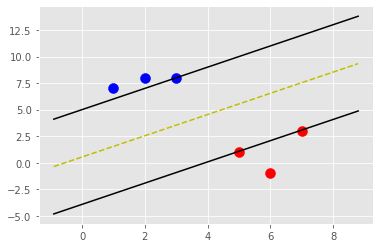1. svm.predict([3,8])
OUTPUT

(-1.0, -1.000000000000098)

### 2. Using Sklearn

Let us now take a look at how can we implement SVM using sklearn. In the following example, I have used Social Network data, please find it attached. I have taken the code reference from the repository.
1. # Importing the libraries
2.
3. import numpy as np
4. import matplotlib.pyplot as plt
5. import pandas as pd
6.
7. # Importing the datasets
8.
10. X = datasets.iloc[:, [2,3]].values
11. Y = datasets.iloc[:, 4].values
12.
13. # Splitting the dataset into the Training set and Test set
14.
15. from sklearn.model_selection import train_test_split
16. X_Train, X_Test, Y_Train, Y_Test = train_test_split(X, Y, test_size = 0.25, random_state = 0)
17.
18. # Feature Scaling
19.
20. from sklearn.preprocessing import StandardScaler
21. sc_X = StandardScaler()
22. X_Train = sc_X.fit_transform(X_Train)
23. X_Test = sc_X.transform(X_Test)
24.
25. # Fitting the classifier into the Training set
26.
27. from sklearn.svm import SVC
28. classifier = SVC(kernel = 'linear', random_state = 0)
29. classifier.fit(X_Train, Y_Train)
30.
31. # Predicting the test set results
32.
33. Y_Pred = classifier.predict(X_Test)
34.
35. # Making the Confusion Matrix
36.
37. from sklearn.metrics import confusion_matrix
38. cm = confusion_matrix(Y_Test, Y_Pred)
39.
40. # Visualising the Training set results
41.
42. from matplotlib.colors import ListedColormap
43. X_Set, Y_Set = X_Train, Y_Train
44. X1, X2 = np.meshgrid(np.arange(start = X_Set[:, 0].min() - 1, stop = X_Set[:, 0].max() + 1, step = 0.01),
45.                      np.arange(start = X_Set[:, 1].min() - 1, stop = X_Set[:, 1].max() + 1, step = 0.01))
46. plt.contourf(X1, X2, classifier.predict(np.array([X1.ravel(), X2.ravel()]).T).reshape(X1.shape),
47.              alpha = 0.75, cmap = ListedColormap(('red''green')))
48. plt.xlim(X1.min(), X1.max())
49. plt.ylim(X2.min(), X2.max())
50. for i, j in enumerate(np.unique(Y_Set)):
51.     plt.scatter(X_Set[Y_Set == j, 0], X_Set[Y_Set == j, 1],
52.                 c = ListedColormap(('red''green'))(i), label = j)
53. plt.title('Support Vector Machine (Training set)')
54. plt.xlabel('Age')
55. plt.ylabel('Estimated Salary')
56. plt.legend()
57. plt.show()
58.
59. # Visualising the Test set results
60.
61. from matplotlib.colors import ListedColormap
62. X_Set, Y_Set = X_Test, Y_Test
63. X1, X2 = np.meshgrid(np.arange(start = X_Set[:, 0].min() - 1, stop = X_Set[:, 0].max() + 1, step = 0.01),
64.                      np.arange(start = X_Set[:, 1].min() - 1, stop = X_Set[:, 1].max() + 1, step = 0.01))
65. plt.contourf(X1, X2, classifier.predict(np.array([X1.ravel(), X2.ravel()]).T).reshape(X1.shape),
66.              alpha = 0.75, cmap = ListedColormap(('red''green')))
67. plt.xlim(X1.min(), X1.max())
68. plt.ylim(X2.min(), X2.max())
69. for i, j in enumerate(np.unique(Y_Set)):
70.     plt.scatter(X_Set[Y_Set == j, 0], X_Set[Y_Set == j, 1],
71.                 c = ListedColormap(('red''green'))(i), label = j)
72. plt.title('Linear Support Vector Machine (Test set)')
73. plt.xlabel('Age')
74. plt.ylabel('Estimated Salary')
75. plt.legend()
76. plt.show()
77.
78. from sklearn.svm import SVC
79. classifier = SVC(kernel = 'rbf', random_state = 0)
80. classifier.fit(X_Train, Y_Train)
81.
82. # Predicting the test set results
83.
84. Y_Pred = classifier.predict(X_Test)
85.
86. # Making the Confusion Matrix
87.
88. from sklearn.metrics import confusion_matrix
89. cm = confusion_matrix(Y_Test, Y_Pred)
90.
91. # Visualising the Test set results
92.
93. from matplotlib.colors import ListedColormap
94. X_Set, Y_Set = X_Test, Y_Test
95. X1, X2 = np.meshgrid(np.arange(start = X_Set[:, 0].min() - 1, stop = X_Set[:, 0].max() + 1, step = 0.01),
96.                      np.arange(start = X_Set[:, 1].min() - 1, stop = X_Set[:, 1].max() + 1, step = 0.01))
97. plt.contourf(X1, X2, classifier.predict(np.array([X1.ravel(), X2.ravel()]).T).reshape(X1.shape),
98.              alpha = 0.75, cmap = ListedColormap(('red''green')))
99. plt.xlim(X1.min(), X1.max())
100. plt.ylim(X2.min(), X2.max())
101. for i, j in enumerate(np.unique(Y_Set)):
102.     plt.scatter(X_Set[Y_Set == j, 0], X_Set[Y_Set == j, 1],
103.                 c = ListedColormap(('red''green'))(i), label = j)
104. plt.title('Radial Basis Function (RBF) Support Vector Machine (Test set)')
105. plt.xlabel('Age')
106. plt.ylabel('Estimated Salary')
107. plt.legend()
108. plt.show()
OUTPUT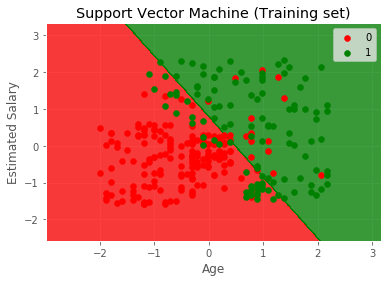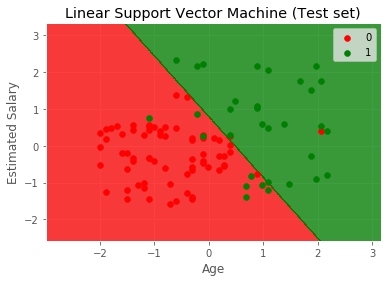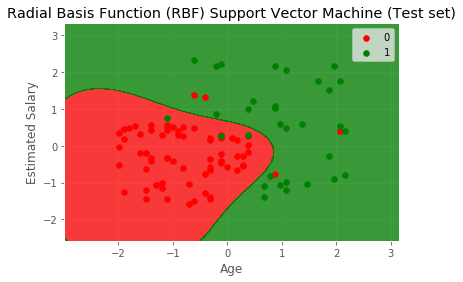### 3. Using Tensorflow

Let us now take a look at how can we implement SVM using TensorFlow. In the following example, I am using the IRIS dataset. I have taken the code reference from the repository.

Note: tf.disable_v2_behaviour() is used to use the Tensorflow 1 functionalities, as i have Tensorflow 2 installed on my PC.
1. import matplotlib.pyplot as plt
2. import numpy as np
3. import tensorflow.compat.v1 as tf
4. tf.disable_v2_behavior()
5. from sklearn import datasets
6. from tensorflow.python.framework import ops
7. ops.reset_default_graph()
8.
9. # Set random seeds
10. np.random.seed(7)
11. tf.set_random_seed(7)
12.
13. # Create graph
14. sess = tf.Session()
15.
17. # iris.data = [(Sepal Length, Sepal Width, Petal Length, Petal Width)]
19. x_vals = np.array([[x, x] for x in iris.data])
20. y_vals = np.array([1 if y == 0 else -1 for y in iris.target])
21.
22. # Split data into train/test sets
23. train_indices = np.random.choice(len(x_vals),
24.                                  int(round(len(x_vals)*0.9)),
25.                                  replace=False)
26. test_indices = np.array(list(set(range(len(x_vals))) - set(train_indices)))
27. x_vals_train = x_vals[train_indices]
28. x_vals_test = x_vals[test_indices]
29. y_vals_train = y_vals[train_indices]
30. y_vals_test = y_vals[test_indices]
31.
32. # Declare batch size
33. batch_size = 135
34.
35. # Initialize placeholders
36. x_data = tf.placeholder(shape=[None2], dtype=tf.float32)
37. y_target = tf.placeholder(shape=[None1], dtype=tf.float32)
38.
39. # Create variables for linear regression
40. A = tf.Variable(tf.random_normal(shape=))
41. b = tf.Variable(tf.random_normal(shape=))
42.
43. # Declare model operations
44. model_output = tf.subtract(tf.matmul(x_data, A), b)
45.
46. # Declare vector L2 'norm' function squared
47. l2_norm = tf.reduce_sum(tf.square(A))
48.
49. # Declare loss function
50. # Loss = max(0, 1-pred*actual) + alpha * L2_norm(A)^2
51. # L2 regularization parameter, alpha
52. alpha = tf.constant([0.01])
53. # Margin term in loss
54. classification_term = tf.reduce_mean(tf.maximum(0., tf.subtract(1., tf.multiply(model_output, y_target))))
55. # Put terms together
56. loss = tf.add(classification_term, tf.multiply(alpha, l2_norm))
57.
58. # Declare prediction function
59. prediction = tf.sign(model_output)
60. accuracy = tf.reduce_mean(tf.cast(tf.equal(prediction, y_target), tf.float32))
61.
62. # Declare optimizer
64. train_step = my_opt.minimize(loss)
65.
66. # Initialize variables
67. init = tf.global_variables_initializer()
68. sess.run(init)
69.
70. # Training loop
71. loss_vec = []
72. train_accuracy = []
73. test_accuracy = []
74. for i in range(500):
75.     rand_index = np.random.choice(len(x_vals_train), size=batch_size)
76.     rand_x = x_vals_train[rand_index]
77.     rand_y = np.transpose([y_vals_train[rand_index]])
78.     sess.run(train_step, feed_dict={x_data: rand_x, y_target: rand_y})
79.
80.     temp_loss = sess.run(loss, feed_dict={x_data: rand_x, y_target: rand_y})
81.     loss_vec.append(temp_loss)
82.
83.     train_acc_temp = sess.run(accuracy, feed_dict={
84.         x_data: x_vals_train,
85.         y_target: np.transpose([y_vals_train])})
86.     train_accuracy.append(train_acc_temp)
87.
88.     test_acc_temp = sess.run(accuracy, feed_dict={
89.         x_data: x_vals_test,
90.         y_target: np.transpose([y_vals_test])})
91.     test_accuracy.append(test_acc_temp)
92.
93.     if (i + 1) % 100 == 0:
94.         print('Step #{} A = {}, b = {}'.format(
95.             str(i+1),
96.             str(sess.run(A)),
97.             str(sess.run(b))
98.         ))
99.         print('Loss = ' + str(temp_loss))
100.
101. # Extract coefficients
102. [[a1], [a2]] = sess.run(A)
103. [[b]] = sess.run(b)
104. slope = -a2/a1
105. y_intercept = b/a1
106.
107. # Extract x1 and x2 vals
108. x1_vals = [d[1for d in x_vals]
109.
110. # Get best fit line
111. best_fit = []
112. for i in x1_vals:
113.     best_fit.append(slope*i+y_intercept)
114.
115. # Separate I. setosa
116. setosa_x = [d[1for i, d in enumerate(x_vals) if y_vals[i] == 1]
117. setosa_y = [d[0for i, d in enumerate(x_vals) if y_vals[i] == 1]
118. not_setosa_x = [d[1for i, d in enumerate(x_vals) if y_vals[i] == -1]
119. not_setosa_y = [d[0for i, d in enumerate(x_vals) if y_vals[i] == -1]
120.
121. # Plot data and line
122. plt.plot(setosa_x, setosa_y, 'o', label='I. setosa')
123. plt.plot(not_setosa_x, not_setosa_y, 'x', label='Non-setosa')
124. plt.plot(x1_vals, best_fit, 'r-', label='Linear Separator', linewidth=3)
125. plt.ylim()
126. plt.legend(loc='lower right')
127. plt.title('Sepal Length vs Petal Width')
128. plt.xlabel('Petal Width')
129. plt.ylabel('Sepal Length')
130. plt.show()
131.
132. # Plot train/test accuracies
133. plt.plot(train_accuracy, 'k-', label='Training Accuracy')
134. plt.plot(test_accuracy, 'r--', label='Test Accuracy')
135. plt.title('Train and Test Set Accuracies')
136. plt.xlabel('Generation')
137. plt.ylabel('Accuracy')
138. plt.legend(loc='lower right')
139. plt.show()
140.
141. # Plot loss over time
142. plt.plot(loss_vec, 'k-')
143. plt.title('Loss per Generation')
144. plt.xlabel('Generation')
145. plt.ylabel('Loss')
146. plt.show()
OUTPUT

Step #100 A = [[-0.4810509 ][ 0.05859518]], b = [[-1.8697345]]Loss = [0.64420575]
Step #200 A = [[-0.4076391 ][-0.25413615]], b = [[-1.9181045]]Loss = [0.45963168]
Step #300 A = [[-0.34309638][-0.55148035]], b = [[-1.9694378]]Loss = [0.34777495]
Step #400 A = [[-0.28505743][-0.83066034]], b = [[-2.023808]]Loss = [0.25850892]
Step #500 A = [[-0.22314341][-1.096483 ]], b = [[-2.0792139]]Loss = [0.2473848]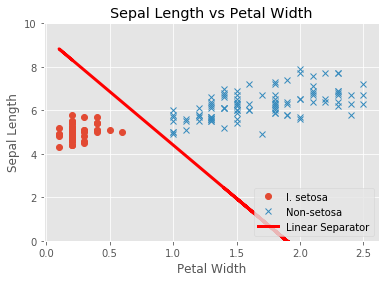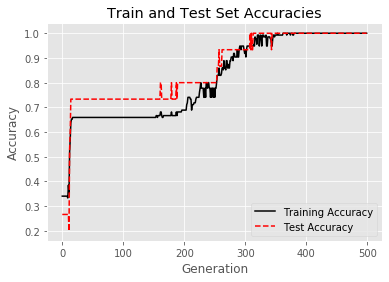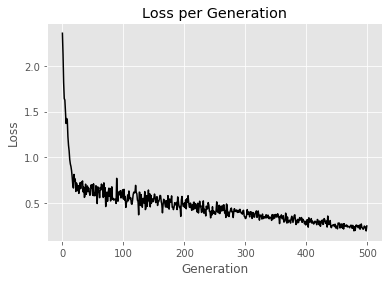## Conclusion

In this chapter, we studied the support vector machine.

With this, we are done with learning machine learning algorithms. Now its time to do some hands-on. So from the next chapter onwards, we will do some projects.
Next » Machine Leaning Project 1: Housing Price Prediction